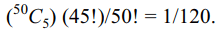## Probability Questions and Answers Part-10

1. If A and B are two events, then which one of the following is not always true
a) $P \left(A \cap B\right)\leq P\left(A\right)+P\left(B\right)-1$
b) $P \left(A \cap B\right)\leq P\left(A\right)$
c) $P \left(A' \cap B'\right)\leq P\left(A'\right)+P\left(B'\right)-1$
d) $P \left(A \cap B\right)= P\left(A\right)P\left(B\right)$

Explanation: (d) is true if and only if A and B are independent

2. A sum of money is rounded off to the nearest rupee. The probability that the round off error is at most 10 paise is
a) 1/10
b) 11/100
c) 3/25
d) 21/100

Explanation:3. The probability that an electric bulb will last 150 days or more is 0.7 and that it will last at most 160 days is 0.8. The probability that the bulb will last 150 to 160 days is
a) 0.5
b) 1/3
c) 0.56
d) 0.59

Explanation:4. A bag contains three tickets numbered 1, 2 and 3. A ticket is drawn at random and put back in the bag, and this is done four times. The probability that the sum of the numbers drawn is even is
a) 40/81
b) 41/81
c) 14/27
d) 13/81

Explanation: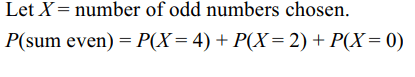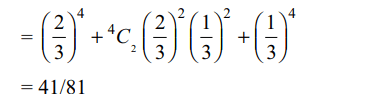5. Two numbers x and y are selected at random from the set {1, 2, 3,…, 3N}. The probability that x2 – y2 is divisible by 3 is
a) (3N – 1)/3N
b) (N – 1)/N
c) (5N – 3)/(9N – 3)
d) (N – 1)/2N

Explanation:6. Three persons A, B and C are to speak at a function along with 7 other persons. The probability that A speaks before B and B speaks before C is
a) 3/70
b) 1/6
c) 3/7
d) 1/9

Explanation: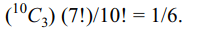7. m men and n women are seated at round table in random order. The probalility that they can be divided into n non-interrecting pairs so that each pair consists of a man and a woman is
a) 1/2n
b) $2\left(2^{n}-1\right)/^{2n}C_{n}$
c) $2n/^{2n}C_{n}$
d) $1/\left(^{n}C_{n}\right)^{2}$

Explanation: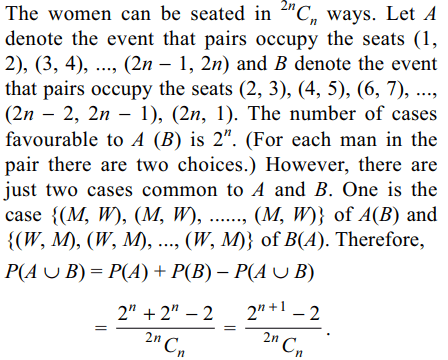8. If a is an integer lying in [– 5, 30], then the probability that the graph of $y = x^{2} + 2 \left(a + 4\right) x –5a + 64$       is strictly above the x – axis is
a) 1/6
b) 7/36
c) 2/9
d) 3/5

Explanation: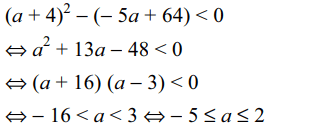9. Two numbers X and Y are chosen at random (without replacement) from the set {1, 2, 5N}. The probability that $X^{4} -Y^{4}$  is divisible by 5 is
a) $\frac{N-1}{5N-1}$
b) $\frac{4\left(4N-1\right)}{5\left(5N-1\right)}$
c) $\frac{17N-5}{5\left(5N-1\right)}$
d) $\frac{3N-5}{5\left(5N-1\right)}$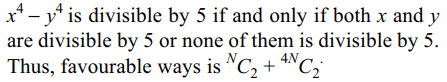b) $1/5^{50}$
c) $1/50^{5}$